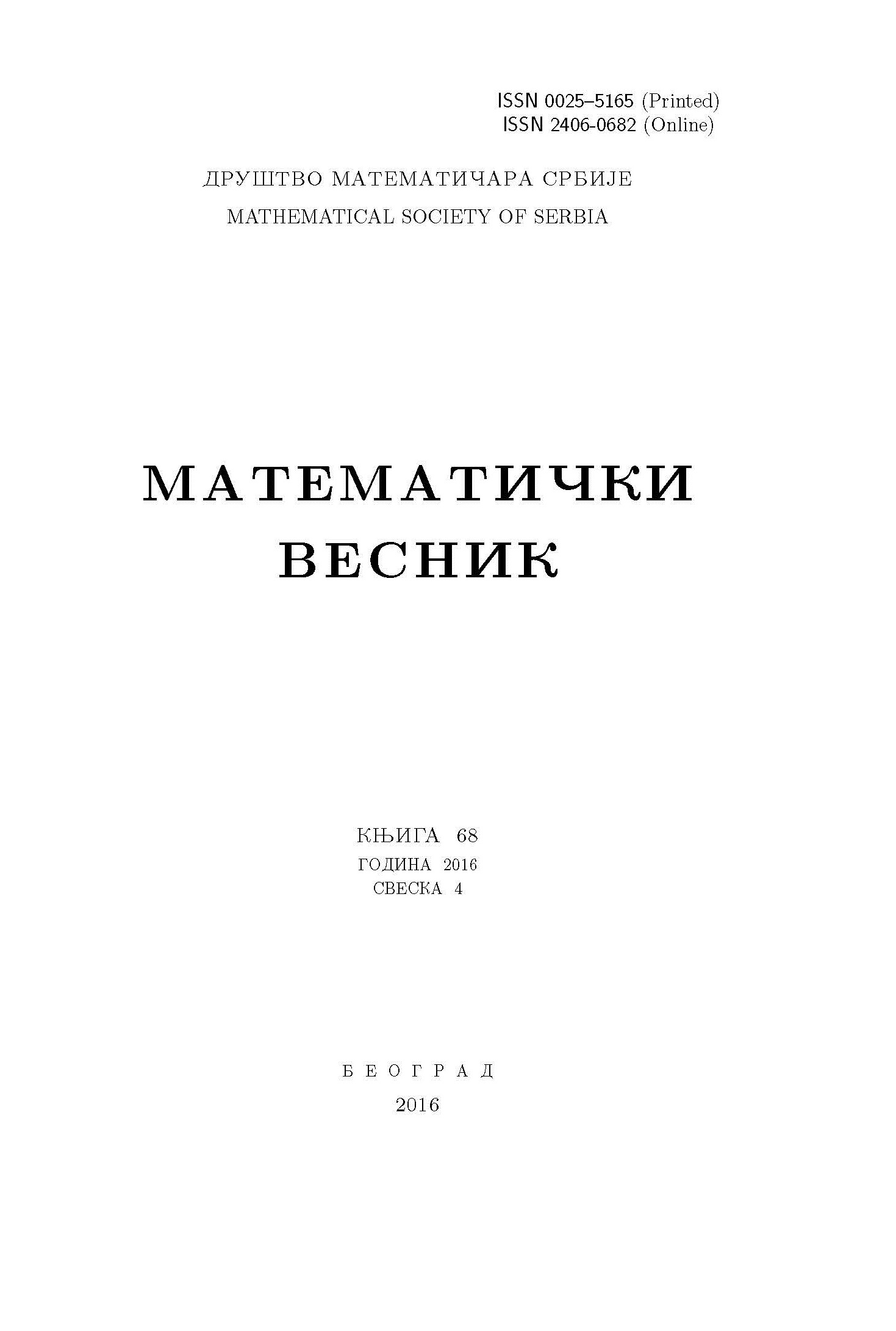﻿ Matematički Vesnik ﻿
 MATEMATIČKI VESNIK МАТЕМАТИЧКИ ВЕСНИКEXISTENCE OF ONE WEAK SOLUTION FOR ELLIPTIC EQUATIONS INVOLVING A GENERAL OPERATOR IN DIVERGENCE FORM S. Amirkhanlou, M. K. Moghadam, Y. Khalili AbstractIn this article, we establish the existence of at least one non-trivial classical solution for a class of elliptic equations involving a general operator in divergence form, subject to Dirichlet boundary conditions in a smooth bounded domain in $\mathbb{R}^N$. A critical point result for differentiable functionals is discussed. Our technical approach is based on variational methods. In addition, an example to illustrate our results is given.Keywords: Existence result; weak solution; divergence type equations; variational methods; critical point theory. MSC: 35J35, 35J60 Pages:  1$-$9

﻿